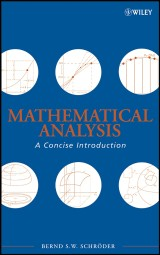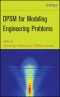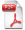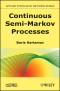# Details## Mathematical Analysis

A Concise Introduction
1. Aufl.
 114,99 € Verlag: Wiley Format: PDF Veröffentl.: 28.01.2008 ISBN/EAN: 9780470226766 Sprache: englisch Anzahl Seiten: 592

DRM-geschütztes eBook, Sie benötigen z.B. Adobe Digital Editions und eine Adobe ID zum Lesen.

## Beschreibungen

#### Titelbeschreibung

A self-contained introduction to the fundamentals of mathematical analysis<br /> <br /> Mathematical Analysis: A Concise Introduction presents the foundations of analysis and illustrates its role in mathematics. By focusing on the essentials, reinforcing learning through exercises, and featuring a unique "learn by doing" approach, the book develops the reader's proof writing skills and establishes fundamental comprehension of analysis that is essential for further exploration of pure and applied mathematics. This book is directly applicable to areas such as differential equations, probability theory, numerical analysis, differential geometry, and functional analysis.<br /> <br /> Mathematical Analysis is composed of three parts:<br /> <br /> ?Part One presents the analysis of functions of one variable, including sequences, continuity, differentiation, Riemann integration, series, and the Lebesgue integral. A detailed explanation of proof writing is provided with specific attention devoted to standard proof techniques. To facilitate an efficient transition to more abstract settings, the results for single variable functions are proved using methods that translate to metric spaces.<br /> <br /> ?Part Two explores the more abstract counterparts of the concepts outlined earlier in the text. The reader is introduced to the fundamental spaces of analysis, including Lp spaces, and the book successfully details how appropriate definitions of integration, continuity, and differentiation lead to a powerful and widely applicable foundation for further study of applied mathematics. The interrelation between measure theory, topology, and differentiation is then examined in the proof of the Multidimensional Substitution Formula. Further areas of coverage in this section include manifolds, Stokes' Theorem, Hilbert spaces, the convergence of Fourier series, and Riesz' Representation Theorem.<br /> <br /> ?Part Three provides an overview of the motivations for analysis as well as its applications in various subjects. A special focus on ordinary and partial differential equations presents some theoretical and practical challenges that exist in these areas. Topical coverage includes Navier-Stokes equations and the finite element method.<br /> <br /> Mathematical Analysis: A Concise Introduction includes an extensive index and over 900 exercises ranging in level of difficulty, from conceptual questions and adaptations of proofs to proofs with and without hints. These opportunities for reinforcement, along with the overall concise and well-organized treatment of analysis, make this book essential for readers in upper-undergraduate or beginning graduate mathematics courses who would like to build a solid foundation in analysis for further work in all analysis-based branches of mathematics.

#### Inhaltsverzeichnis

<p>Preface xi</p> <p><b>Part I: Analysis of Functions of a Single Real Variable</b></p> <p><b>1 The Real Numbers 1</b></p> <p>1.1 Field Axioms 1</p> <p>1.2 Order Axioms 4</p> <p>1.3 Lowest Upper and Greatest Lower Bounds 8</p> <p>1.4 Natural Numbers, Integers, and Rational Numbers 11</p> <p>1.5 Recursion, Induction, Summations, and Products 17</p> <p><b>2 Sequences of Real Number V 25</b></p> <p>2.1 Limits 25</p> <p>2.2 Limit Laws 30</p> <p>2.3 Cauchy Sequences 36</p> <p>2.4 Bounded Sequences 40</p> <p>2.5 Infinite Limits 44</p> <p><b>3 Continuous Functions 49</b></p> <p>3.1 Limits of Functions 49</p> <p>3.2. Limit Laws 52</p> <p>3.3 One-Sided Limits and Infinite Limits 56</p> <p>3.4 Continuity 59</p> <p>3.5 Properties of Continuous Functions 66</p> <p>3.6 Limits at Infinity 69</p> <p><b>4 Differentiable Functions 71</b></p> <p>4.1 Differentiability 71</p> <p>4.2 Differentiation Rules 74</p> <p>4.3 Rolle's Theorem and the Mean Value Theorem 80</p> <p><b>5 The Riemann Integral I 85</b></p> <p>5.1 Riemann Sums and the Integral 85</p> <p>5.2 Uniform Continuity and Integrability of Continuous Functions 91</p> <p>5.3 The Fundamental Theorem of Calculus 95</p> <p>5.4 The Darboux Integral 97</p> <p><b>6 Series of Real Numbers I 101</b></p> <p>6.1 Series as a Vehicle to Define Infinite Sums 101</p> <p>6.2 Absolute Convergence and Unconditional Convergence 108</p> <p><b>7 Some Set Theory 117</b></p> <p>7.1 The Algebra of Sets 117</p> <p>7.2 Countable Sets 122</p> <p>7.3 Uncountable Sets 124</p> <p><b>8 The Riemann Integral II 127</b></p> <p>8.1 Outer Lebesgue Measure 127</p> <p>8.2 Lebesgue's Criterion for Riemann Integrability 131</p> <p>8.3 More Integral Theorems 136</p> <p>8.4 Improper Riemann Integrals 140</p> <p><b>9 The Lebesgue Integral 145</b></p> <p>9.1 Lebesgue Measurable Sets 147</p> <p>9.2 Lebesgue Measurable Functions 153</p> <p>9.3 Lebesgue Integration 158</p> <p>9.4 Lebesgue Integrals versus Riemann Integrals 165</p> <p><b>10 Series of Real Numbers II 169</b></p> <p>10.1 Limits Superior and Inferior 169</p> <p>10.2 The Root Test and the Ratio Test 172</p> <p>10.3 Power Series 175</p> <p><b>11 Sequences of Functions 179</b></p> <p>11.1 Notions of Convergence 179</p> <p>11.2 Uniform Convergence 182</p> <p><b>12 Transcendental Functions 189</b></p> <p>12.1 The Exponential Function 189</p> <p>12.2 Sine and Cosine 193</p> <p>12.3 L.' H&ocirc;pital's Rule 199</p> <p><b>13 Numerical Methods 203</b></p> <p>13.1 Approximation with Taylor Polynomials 204</p> <p>13.2 Newton's Method 208</p> <p>13.3 Numerical Integration 214</p> <p><b>Part II: Analysis in Abstract Spaces</b></p> <p><b>14 Integration on Measure Spaces 225</b></p> <p>14.1 Measure Spaces 225</p> <p>14.2 Outer Measures 230</p> <p>14.3 Measurable Functions 234</p> <p>14.4 Integration of Measurable Functions 235</p> <p>14.5 Monotone and Dominated Convergence 238</p> <p>14.6 Convergence in Mean, in Measure, and Almost Everywhere 242</p> <p>14.7 Product Ϭ-Algebras 245</p> <p>14.8 Product Measures and Fubini's Theorem 251</p> <p><b>15 The Abstract Venues for Analysis 255</b></p> <p>15.1 Abstraction I: Vector Spaces 255</p> <p>15.2 Representation of Elements; Bases and Dimension 259</p> <p>15.3 Identification of Spaces: Isomorphism 262</p> <p>15.4 Abstraction II: Inner Product Spaces 264</p> <p>15.5 Nicer Representations: Orthonormal Sets 267</p> <p>15.6 Abstraction III: Norrned Spaces 269</p> <p>15.7 Abstraction IV: Metric Spaces 275</p> <p>15.8 <i>L<sup>P</sup></i> Spaces 278</p> <p>15.9 Another Number Field: Complex Numbers 281</p> <p><b>16 The Topology of Metric Spaces 287</b></p> <p>16.1 Convergence of Sequences 287</p> <p>16.2 Completeness 291</p> <p>16.3 Continuous Functions 296</p> <p>16.4 Open and Closed Sets 301</p> <p>16.5 Compactness 309</p> <p>16.6 The Normed Topology of R<i><sup>d</sup></i> 316</p> <p>16.7 Dense Subspaces 322</p> <p>16.8 Connectedness 330</p> <p>16.9 Locally Compact Spaces 333</p> <p><b>17 Differentiation in Normed Spaces 341</b></p> <p>17.1 Continuous Linear Functions 342</p> <p>17.2 Matrix Representation of Linear Functions 348</p> <p>17.3 Differentiability 353</p> <p>17.4 The Mean Value Theorem 360</p> <p>17.5 How Partial Derivatives Fit In 362</p> <p>17.6 Multilinear Functions (Tensors) 369</p> <p>17.7 Higher Derivatives 373</p> <p>17.8 The. Implicit Function Theorem 380</p> <p><b>18 Measure, Topology, and Differentiation 385</b></p> <p>18.1 Lebesgue Measurable Sets in R<i><sup>d</sup></i> 385</p> <p>18.2 C<sup>ꝏ</sup> and Approximation of Integrable Functions 391</p> <p>18.3 Tensor Algebra and Determinants 397</p> <p>18.4 Multidimensional Substitution 407</p> <p><b>19 Introduction to Differential Geometry 421</b></p> <p>19.1 Manifolds 421</p> <p>19.2 Tangent Spaces and Differentiable Functions 427</p> <p>19.3 Differential Forms, Integrals Over the Unit Cube 434</p> <p>19.4 <i>k</i>-Forms and Integrals Over <i>k</i>-Chains 443</p> <p>19.5 Integration on Manifolds 452</p> <p>19.6 Stokes' Theorem 458</p> <p><b>20 Hilbert Spaces 463</b></p> <p>20.1 Orthonormal Bases 463</p> <p>20.2 Fourier Series 467</p> <p>20.3 The Riesz Representation Theorem 475</p> <p><b>Part III: Applied Analysis</b></p> <p><b>21 Physics Background 483</b></p> <p>21.1 Harmonic Oscillators 484</p> <p>21.2 Heat and Diffusion 486</p> <p>21.3 Separation of Variables, Fourier Series, and Ordinary Differential Equa-tions 490</p> <p>21.4 Maxwell's Equations 493</p> <p>21.5 The Navier Stokes Equation for the Conservation of Mass 496</p> <p><b>22 Ordinary Differential Equations 505</b></p> <p>22.1 Burwell Space Valued Differential Equations 505</p> <p>22.2 An Existence and Uniqueness Theorem 508</p> <p>22.3 Linear Differential Equations 510</p> <p><b>23 The Finite Element Method 513</b></p> <p>23.1 Ritz-Galerkin Approximation 513</p> <p>23.2 Wealth Differentiable Functions 518</p> <p>23,3 Sobolev Spaces 524</p> <p>23.4 Elliptic Differential Operators 532</p> <p>23.5 Finite Elements 536</p> <p>Conclusion and Outlook 544</p> <p><b>Appendices</b></p> <p><b>A Logic 545</b></p> <p>A.1 Statements 545</p> <p>A.2 Negations 546</p> <p><b>B Set Theory 547</b></p> <p>B. 1 The Zermelo-Fraenkel Axioms 547</p> <p>B.2 Relations and Functions 548</p> <p><b>C Natural Numbers, Integers, and Rational Numbers 549</b></p> <p>C.1 The Natural Numbers 549</p> <p>C.2 The Integers 550</p> <p>C.3 The Rational Numbers 550</p> <p>Bibliography 551</p> <p>Index 553</p>

#### Rezension

"This highly original, interesting and very useful book includes over 900 exercises which are ranging in levels of difficulty, from conceptual questions and adaptations of proofs to proofs with and without hints." (<i>Mathematical Reviews</i>, 2008h)

#### Autorenportrait

<b>Bernd S.W. Schroder</b>, PhD, is Edmondson/Crump Professor in the Program of Mathematics and Statistics at Louisiana Tech University. Dr. Schröder is the author of over thirty refereed journal articles on subjects such as ordered sets, probability theory, graph theory, harmonic analysis, computer science, and education. He earned his PhD in mathematics from Kansas State University in 1992.

#### Back cover copy

A self-contained introduction to the fundamentals of mathematical analysis <p>Mathematical Analysis: A Concise Introduction presents the foundations of analysis and illustrates its role in mathematics. By focusing on the essentials, reinforcing learning through exercises, and featuring a unique "learn by doing" approach, the book develops the reader's proof writing skills and establishes fundamental comprehension of analysis that is essential for further exploration of pure and applied mathematics. This book is directly applicable to areas such as differential equations, probability theory, numerical analysis, differential geometry, and functional analysis.</p> <p>Mathematical Analysis is composed of three parts:</p> <p>Part One presents the analysis of functions of one variable, including sequences, continuity, differentiation, Riemann integration, series, and the Lebesgue integral. A detailed explanation of proof writing is provided with specific attention devoted to standard proof techniques. To facilitate an efficient transition to more abstract settings, the results for single variable functions are proved using methods that translate to metric spaces.</p> <p>Part Two explores the more abstract counterparts of the concepts outlined earlier in the text. The reader is introduced to the fundamental spaces of analysis, including Lp spaces, and the book successfully details how appropriate definitions of integration, continuity, and differentiation lead to a powerful and widely applicable foundation for further study of applied mathematics. The interrelation between measure theory, topology, and differentiation is then examined in the proof of the Multidimensional Substitution Formula. Further areas of coverage in this section include manifolds, Stokes' Theorem, Hilbert spaces, the convergence of Fourier series, and Riesz' Representation Theorem.</p> <p>Part Three provides an overview of the motivations for analysis as well as its applications in various subjects. A special focus on ordinary and partial differential equations presents some theoretical and practical challenges that exist in these areas. Topical coverage includes Navier-Stokes equations and the finite element method.</p> <p>Mathematical Analysis: A Concise Introduction includes an extensive index and over 900 exercises ranging in level of difficulty, from conceptual questions and adaptations of proofs to proofs with and without hints. These opportunities for reinforcement, along with the overall concise and well-organized treatment of analysis, make this book essential for readers in upper-undergraduate or beginning graduate mathematics courses who would like to build a solid foundation in analysis for further work in all analysis-based branches of mathematics.</p>

US

## Diese Produkte könnten Sie auch interessieren:DPSM for Modeling Engineering Problems
von: Tribikram Kundu, Dominique Placko144,99 €Continuous Semi-Markov Processes
von: Boris Harlamov157,99 €